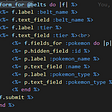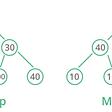# Game of Thrones — I HackerRank Problem

Task :- Given a string, determine whether the letters can be rearranged to form a palindrome. A string is said to be palindrome if it reads the same backward as forward. For eg: madam, racecar.

Approach :- For a string to be palindrome, we can see that every character is present even number of times except the character in the middle(in the case of odd length string). For eg :

• string= ”abcddcba”. The given string is a palindrome and is of even length so every character is present even number of times as every character has its corresponding match in the other half of the string.
• string= “rotator”. This string is a palindrome and is of odd length.Every character is present even number of times except the character ‘a’ in the middle.

Using this property of palindromes. We can determine whether a string can be converted into a palindrome or not.

So we keep count of each letter in the string or in other words the frequency of occurrence of each letter.

Then we determine how many letters are present odd number of times. If there is at most 1 letter that is present odd number of times then string can be converted to palindrome.

— — — — — — — — — — — — — — — — — — — — — — — — — —

Explanation for getting corresponding index:

ASCII value of character ‘a’ is 97, ‘b’ is 98, ‘c’ is 99 and so on.

So we need a mapping to get this range from 0 to 25 (for using 0-based indexing).

If we subtract 97 from ASCII value of any lowercase character we will get the corresponding indexes i.e- 0 for ‘a’, 1 for ‘b’, 2 for ‘c’ and so on till 25 for ‘z’.

— — — — — — — — — — — — — — — — — — — — — — — — — —

Code :-

The time complexity of this approach is linear i.e- O(N) and space complexity is O(1).

Note :- We create an array of 26 integers but space complexity is O(1) because it is not dependent on size/length of the string.

--

--

--

Love podcasts or audiobooks? Learn on the go with our new app.## More from Medium